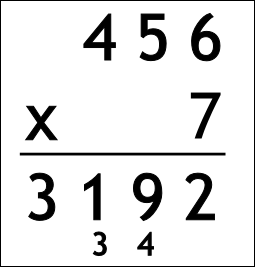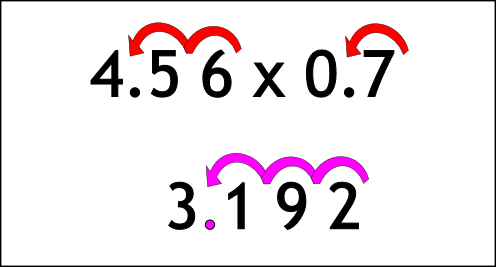The Singing Hedgehog Guide to:
Decimal Multiplication 1

Multiplication is a horizontal process so we:

DO NOT LINE UP THE DECIMAL POINTS!

We MUST NOT put in extra zeroes. Here is an example:

4.56 x 0.7

We start by ignoring the decimal points and just do 456 x 7

If you can't remember how to do this
go to hereNow we must look at the question to see what we did to it.

To change 456 into 4.56 we move the decimal place two times
(or divide by 100)

To change 7 into 0.7
we move the decimal place once
(or divide by 10)We have moved the decimal point THREE times in the question,

so we move the
decimal point THREE times in the answer.